# 0.展示PTA总分

## 0.1.一维数组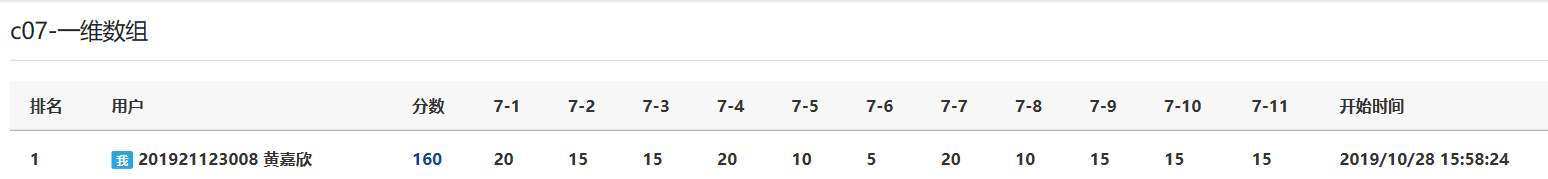## 0.2.二维数组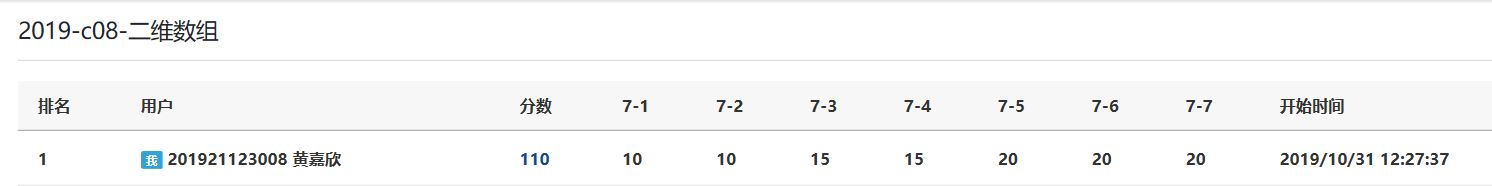## 0.3.字符数组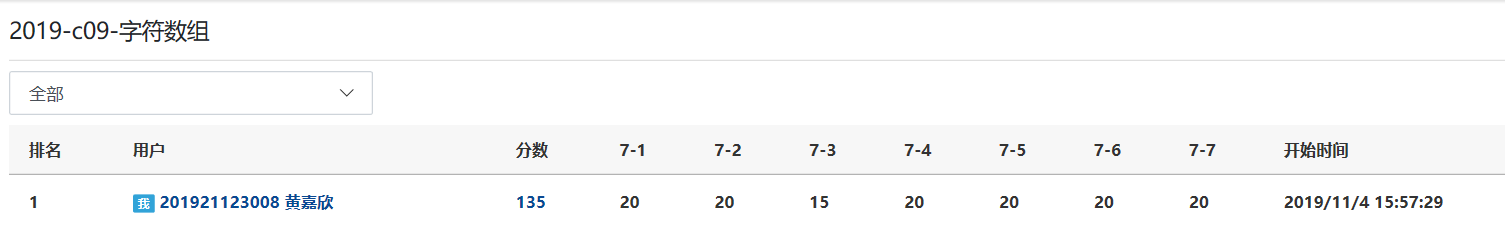# 1.本章学习总结

## 1.1 学习内容总结

#### 一维数组.

• 数组名的命名规则与变量名的命名规则一致
• 常量表达式表示数组元素的个数。可以是常量或符号常量，不能为变量

• int a=
• int a={1,2,3} (未被初始化的后面的元素被系统自动赋值为0）
• int a=

#### 二维数组.

• int a=
• int a={ {1,2,3},{4,5,6} }

#### 字符串数组.

char 数组名[数据长度]

char a={'i','l','o','v','e','y','o','u'}

#### 数组查找数据.

• 遍历数组，用if语句判断数组中的数是否与寻找的数字相等。
• 灵活使用flag，判断有无需要查找的数组。
定义数组变量a用来储存输入10个之内的数字

for int i=0 to 10 do i++
if(a[i]==x) then
flag=1 //找到x，flag重新赋值用以输出找没找到
break //找到X，就跳出循环，减少运行时间
end if
end for
if（flag==1） then
输出“找到了”
else   //flag初始化为0，如果if条件不满足不会进入下面的语句，flag的值一直为0
输出“没找到”
end if


#### 数组插入数据.

• 该题的前提是有序数组（从大到小或者是从小到大），首先找到插入的位置，因为是遍历数组，只要判断插入的数字大于（或者小于）数组中的数字即可，找到插入的位置后，break结束循环。
• 将数组从最后一个数字开始往后挪（不能从插入位置开始往后挪，因为把每个数字往后移一个后，原来该位置上的数字就会被顶替掉，数据会丢失）。
• 再将X赋值给插入的位置。
定义数组变量Num[n]用来储存输入的有序数列

for int i=0 to n do i++
if(a[i]>X) then  //假设该数列是从小到大的排序，如果是从大到小排序的数列，判断条件为a[i]<X
break //找到插入位置就退出循环，这个时候就不在对i做任何运算要确保i为插入数字的位置
end if
end for
for int j=n-1 to i do j-- （这里的i也要进行后移）
a[j+1]=a[j] //因为这里要插入一个数，定义数组的时候尽量大一点，防止越界
end for
a[i]=X //完成后移的循环后，i位置上的数字就空了用来把X放在该位置上



#### 数组删除数据.

• 两个数组删除数据，一个数组储存输入的数据，另一个数组储存删除后的数据。
• 一个数组删除数据，在输入数据的数组中做变换。

定义数组Num1[n]用来储存输入的数字

for int i=0 to n do i++
if(Num1[i]！= X）then
Num2[（int j=0）++]=Num1[i] //把不要删除的数字储存到Num2数组中
end if
end for



定义数组变量a[n]用来储存输入的数字

for int i=0 to n do i++
if（a[i]==X) then //如果数组中的元素有要删除的数字，就把后面的元素依次向前移一个位置，这样就可以把那个需要删除的数字顶掉
for int j=i to n do j++
a[i]=a[i+1]
end for
end if
end for



#### 排序方法.

• 选择法排序：每一次内层循环找到最值，记录最值所在位置的下标，内层循环结束后，交换最值所在的位置和外层循环当前所在的位置。
• 冒泡法排序：相邻两个数字依次做比较，并做交换，每一次内循环的结果就是把最大的数排列在数组的最后面。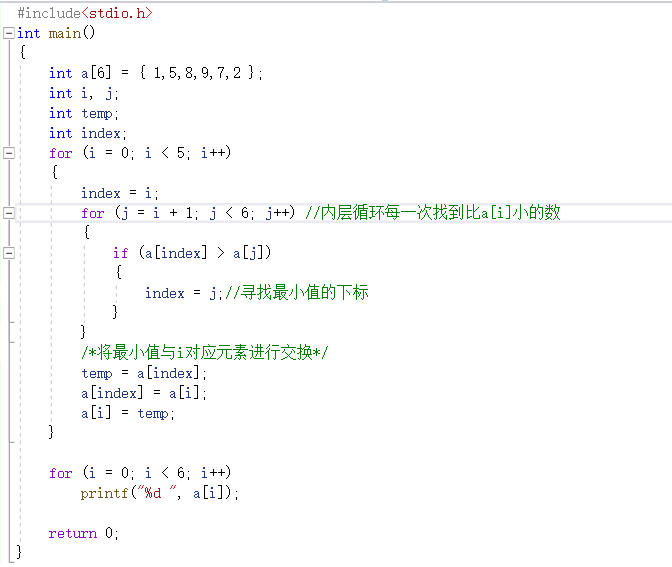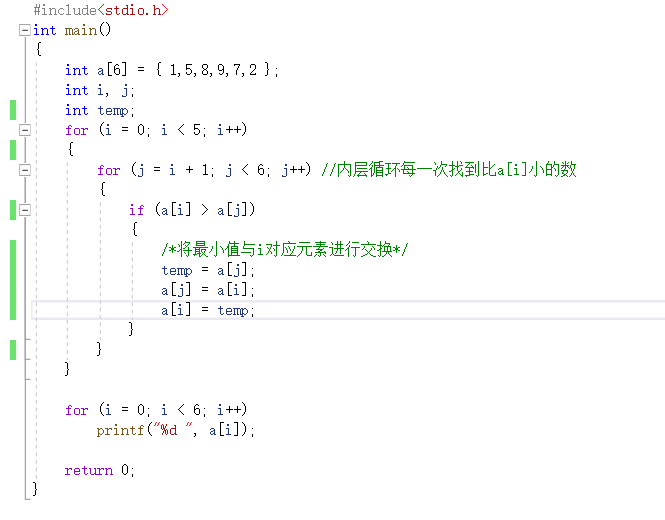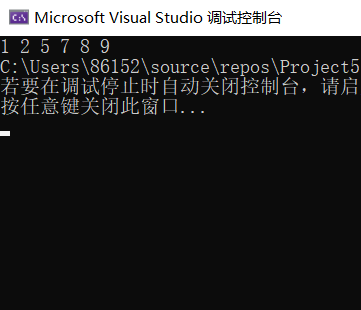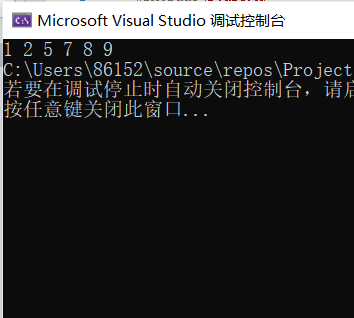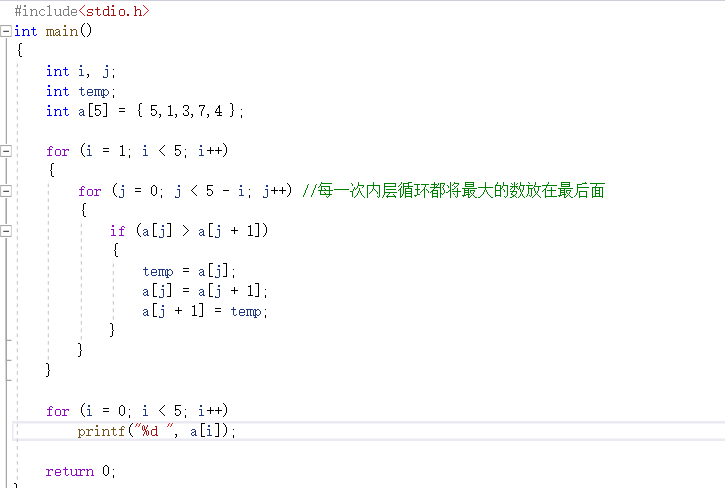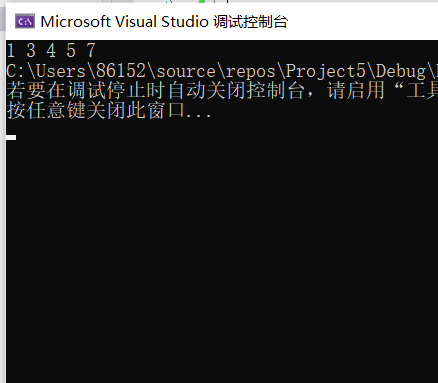#### 数组做枚举用法.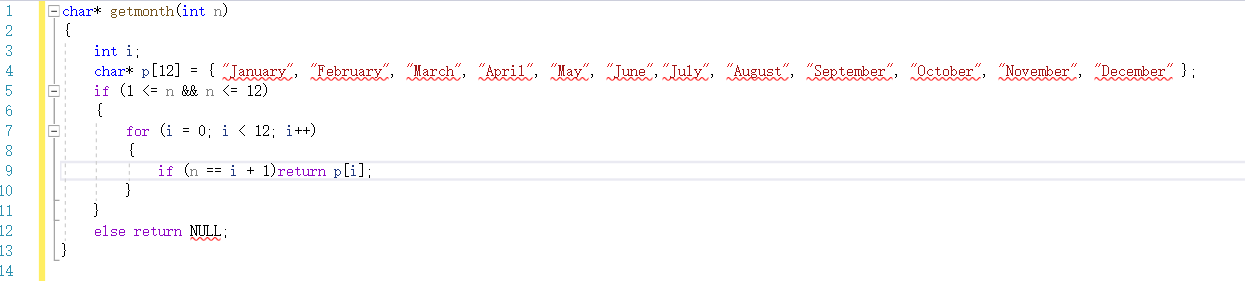(忽略这些红色的线条，VS不支持这个没得法，但是代码本身没有错！！！）

#### 哈希数组.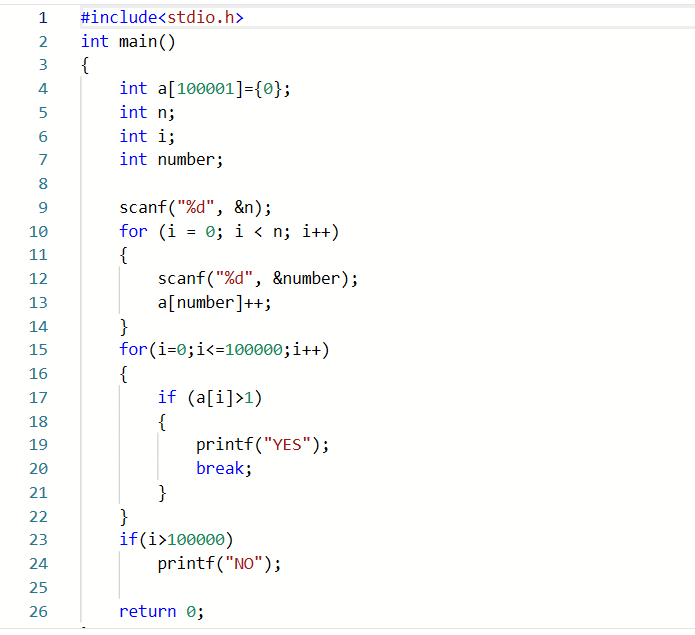## 1.2 本章学习体会

#### 代码量.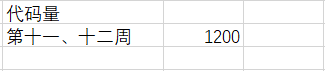# 2.PTA实验作业

## 2.1 一维数组-数组循环左移

### 2.1.1 伪代码

定义一个数组a[n]用来储存输入的序列数字

m=m%n //判断移动位数与输入总个数的关系
if(m==n) then  //移动位数是总个数的整数倍
原样输出"a[n]"数组
else  //输出移动后的数组
for int i=m+1 to n do i++
依次输出数组元素"a[i]"
end for
for int i=0 to m do i++
输出剩下的数组元素"a[i]"
end for
end if


### 2.1.2 代码截图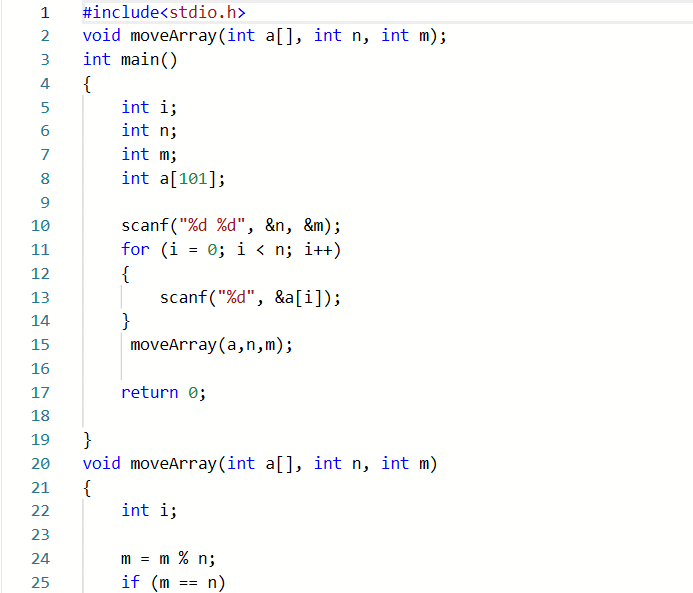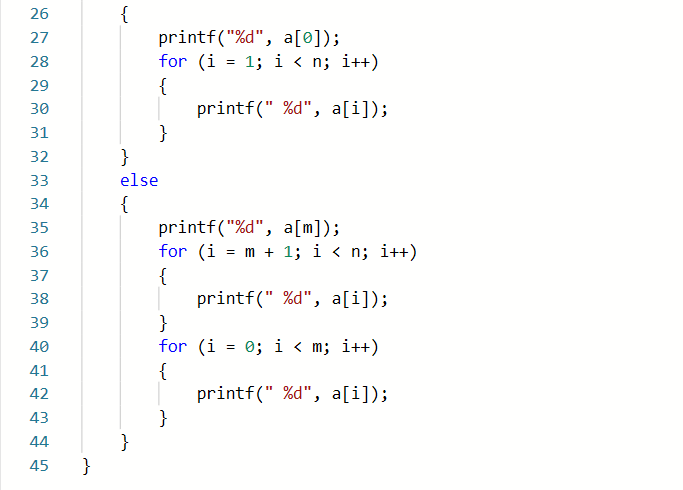### 2.1.3造测试数据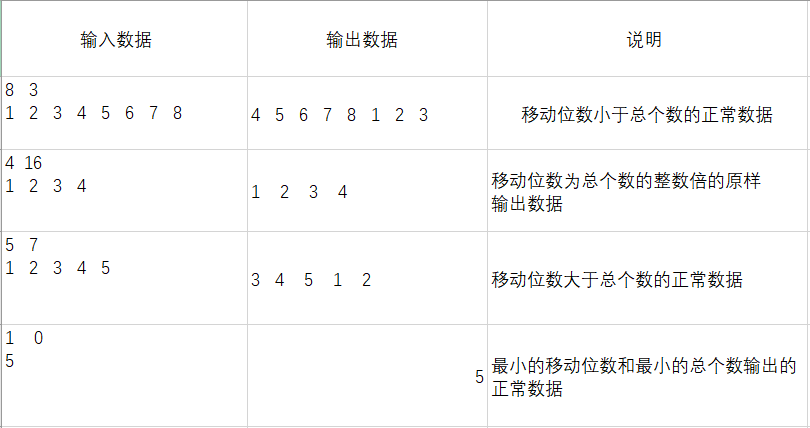### 2.1.4 PTA提交列表及说明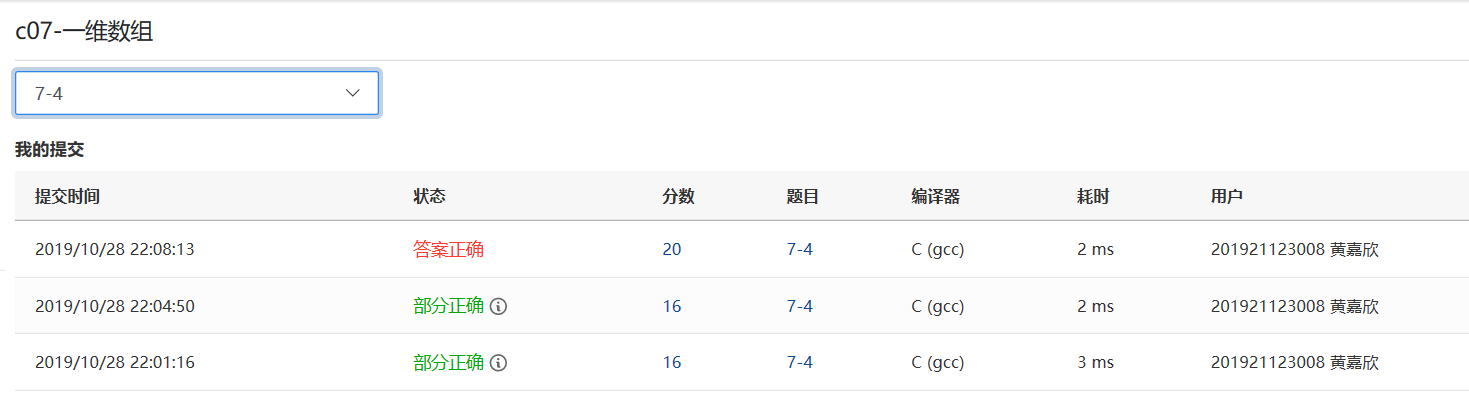Q1：没有考虑到m比n大导致一个测试点过不去。
A1：直接加上m=m%n的表达式后是直接按移动后的数据输出。
Q2：加上Q1的表达式后又忽略是m是n的整数倍的关系报错另一个测试点。
A2：整个函数功能要分为两步，一个是m是n的整数倍关系原样输出，另一个是用取余表达式移动后的数据输出。使用取余表达式可以解决m与n大小的问题。

## 2.1'二维数组-数组循环右移（与左移进行对比）

### 2.1'.1伪代码

定义一个数组a用来储存输入的N阶数组

for int i=0  to n do i++
for int j=0 to n do j++
输入a[i][j]
end for
end for
for i=0 to n do i++
for j=0 to n do j++
b[i][(j+M)%n]=a[i][j] //这里就要求我们找到右移的规律，只要找到规律就很快了，这也是数组的神奇之处！！！
end for
end for
for i=0 to n do i++
for j=0 to n do j++
输出"b[i][j]"
end for
end for


### 2.1'.2代码截图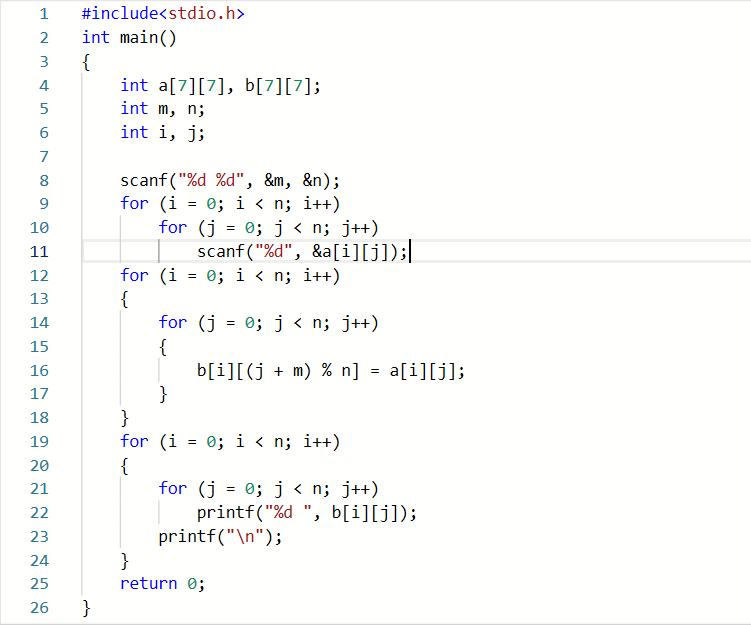## 2.2 二维数组-螺旋方阵

### 2.2.1 伪代码

定义二维数组a用来储存输入的方阵

for int i=0 to 20 do i++
for int j=0 to 20 do j++
b初始化所有元素均为1
end for
end for

for i=0 to n do i++
for j=0 to n dp j++
b[n][n]赋值为0//表明这些元素要填入合适的数字
end for
end for
for i=0 to n do i++
for j=0 to n do j++
b[n][n]赋值为0
end for
end for
while（count< n*n) do
while((int y=0) < n &&b[(int x=0)][y] ==0) do
将t赋值给a[x][y]
令b[x][y]为1//表明a方阵的该元素已经是被成功赋值了
t++;y++;count++;
end
y--;x++
while(y < n &&b[(int x=0)][y] ==0) do
将t赋值给a[x][y]
令b[x][y]为1//表明a方阵的该元素已经是被成功赋值了
t++;x++;count++;
end
x--;y--
while(y >=0 &&b[(int x=0)][y] ==0) do
将t赋值给a[x][y]
令b[x][y]为1//表明a方阵的该元素已经是被成功赋值了
t++;y--;count++;
end
x--;y++;
while(x >=0 &&b[(int x=0)][y] ==0) do
将t赋值给a[x][y]
令b[x][y]为1//表明a方阵的该元素已经是被成功赋值了
t++;x--;count++;
end
x++;y++;
end



### 2.2.2 代码截图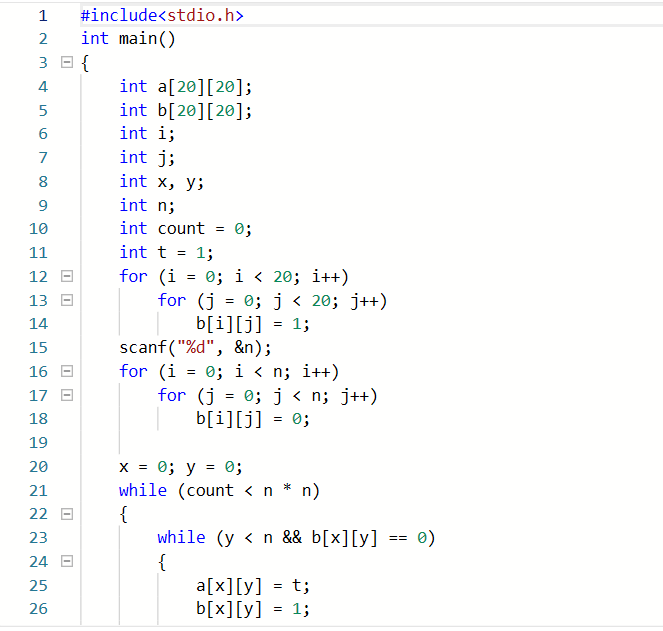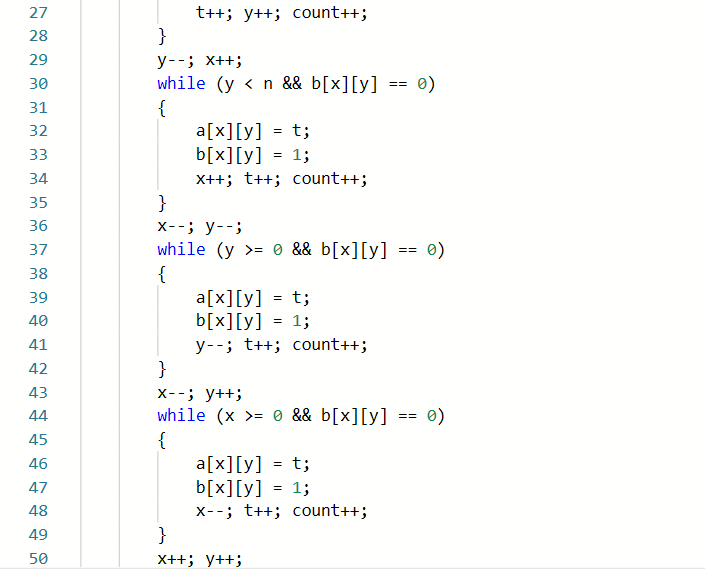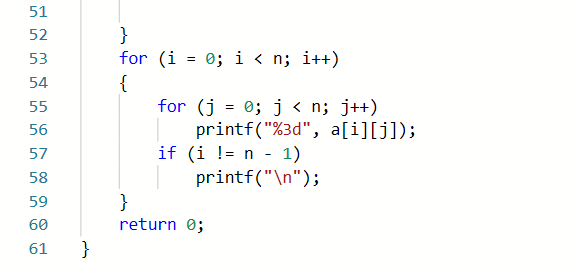### 2.2.3造测试数据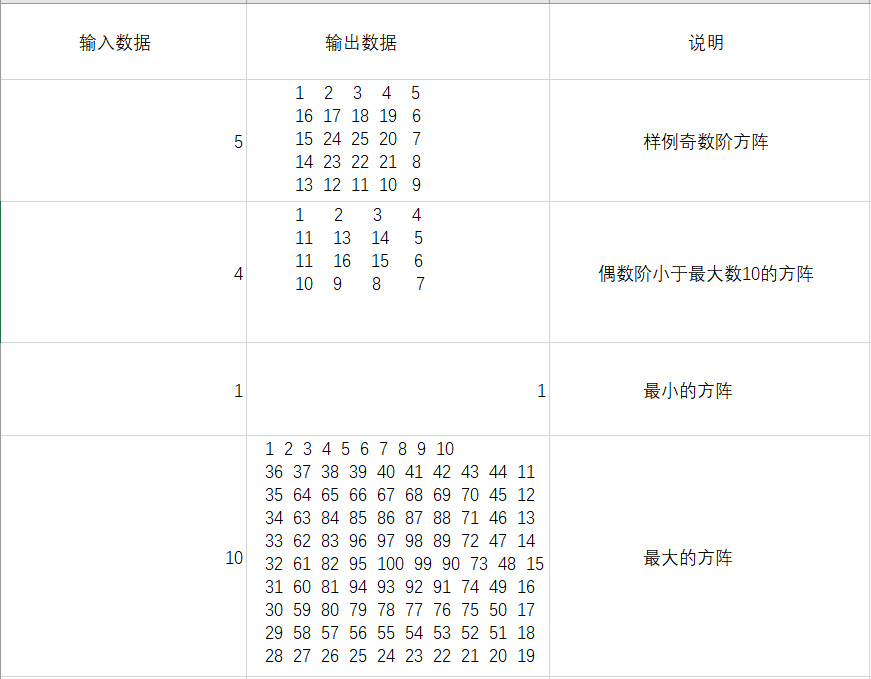### 2.2.4PTA提交列表及说明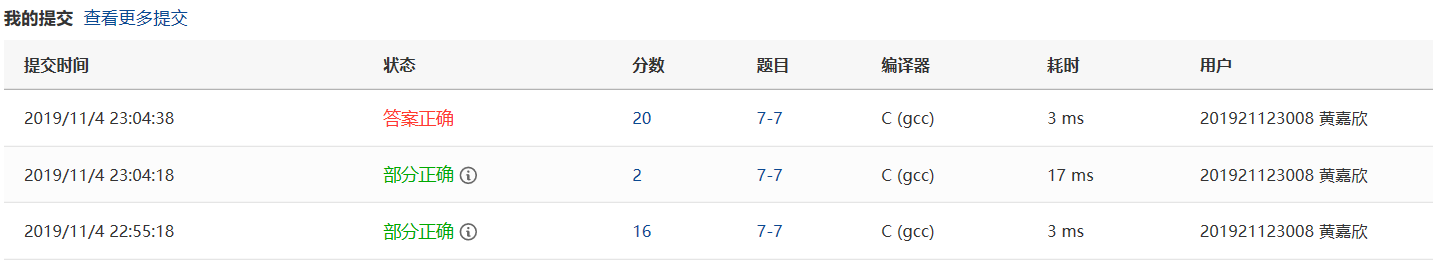Q1:当时思路没有理清楚，输入出来的是行式S形方阵
A1：我当时没找到螺旋方阵的规律，改了一种循环方式，输出来的又是列式S形方阵。
Q2：继A1之后又是一番大改动，可是仍旧输出来的是别的类型方阵，还有一个循环越界了。
A2：经历了N多次这样的大改动循环仍旧无故，然后我只能取百度了，借鉴别的优秀的代码，当时还没怎么明白那个螺旋的规律就开始去折腾自己的代码。
Q3：是的，没错，输出的依然是乱七八糟的方阵，毫不夸张的说--乱七八糟，当时心里急得一批，脑子都炸了......就差撞豆腐了
A3：错了总是要改错的勒，万能的度娘又来了，又是去网上找答案咯，看网上的代码，慢慢的知道螺旋的规律，就自己在纸上罗列了那个规律，慢慢的就把那个代码差不多快写出来了....
.
. 这处省略复杂的调试过程
.

## 2.3字符串数组-大数加法

### 2.3.1伪代码

定义字符串数组a用来储存输入的其中一个相加的大数

if(len1 > len2) then
for int i=0 to len1 do i++
c[i] = c[i] + b1[i] + flag
if(c[i] >= 10)
c[i] = c[i] - 10
flag=1//如果某一位相加超过十，则上一位对应加1
else
flag=0
end if
end for
if ((c[len1 - 1] + b1[len1 - 1]) >= 10) then
输出"1" //最高位超过十，输出1，不往数组里面写元素是怕会越界
end if
for i = len1 -1 to 0 do i--
输出"c[i]"
end for
else
for int i=0 to len2 do i++
c[i] = c[i] + b1[i] + flag
if(c[i] >= 10) then
c[i] = c[i] - 10
flag=1//如果某一位相加超过十，则上一位对应加1
else
flag=0
end if
end for
if ((c[len2 - 1] + b1[len2 - 1]) >= 10)
输出"1" //最高位超过十，输出1，不往数组里面写元素是怕会越界
end if
for i = len2 -1 to 0 do i--
输出"c[i]"
end for
end if


### 2.3.2代码截图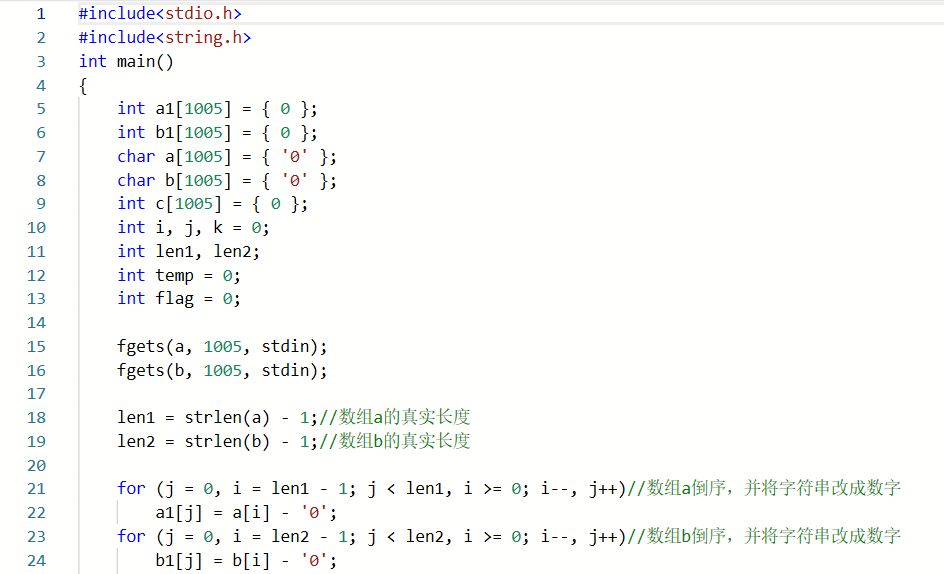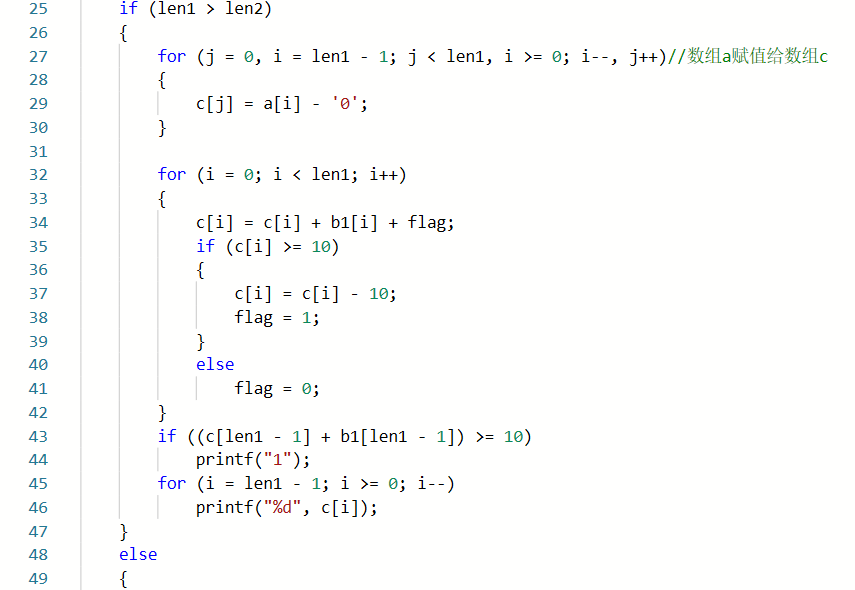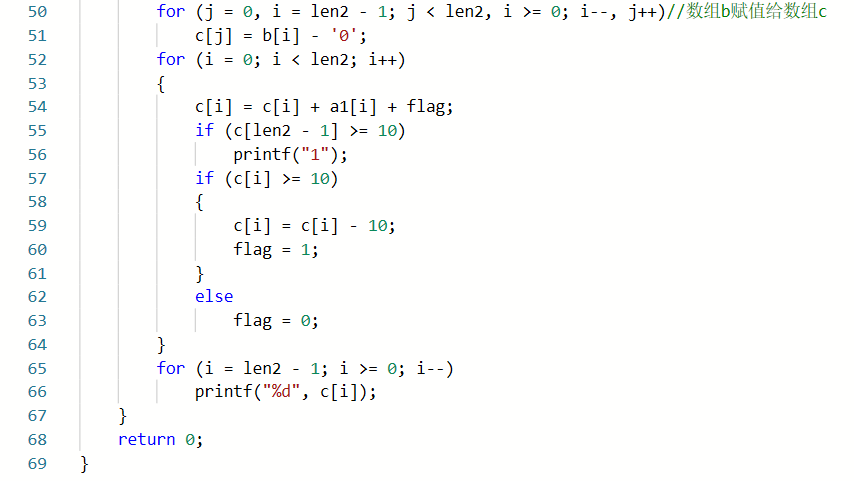### 2.3.3造测试数据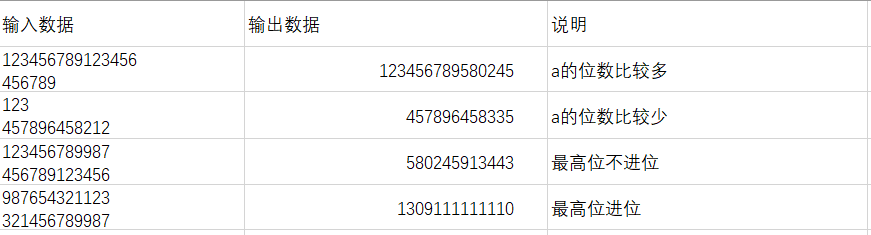### 2.3.4PTA提交列表及说明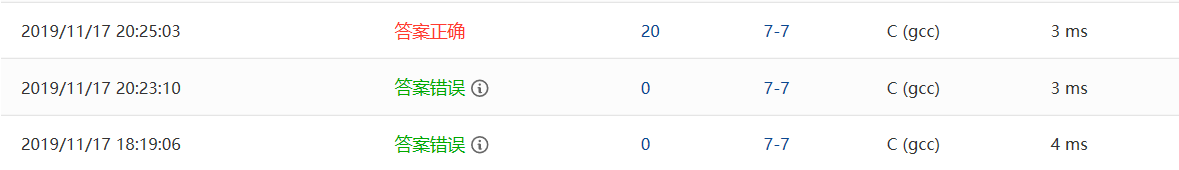Q1：当时那个进位那个地方的代码写错了，一旦有一个进位，之后的每一位数字都要加1
A1：灵活定义一个flag=0；如果进入那个要进位的条件就让它等于1，这样下一组相加就会多加1。不满足进位条件仍旧为0，不影响下一组运算。
Q2：最高位进位的测试点没有考虑完全，我把最高位进位放到那个数组最后一个元素+1里面了
A2：如果加1就要放到数组中去，有可能会越界，之前没有考虑到这一方面的问题，所以在输出之前加一个条件，如果最高位也要进位，则另外输出1

# 3.阅读代码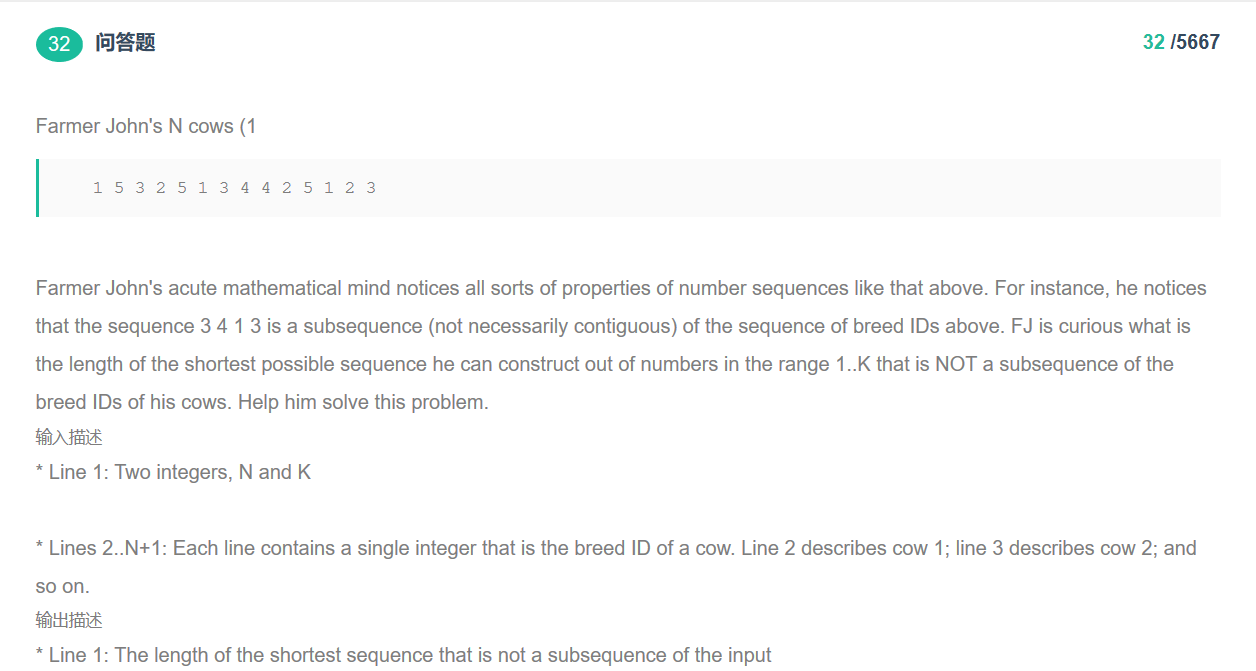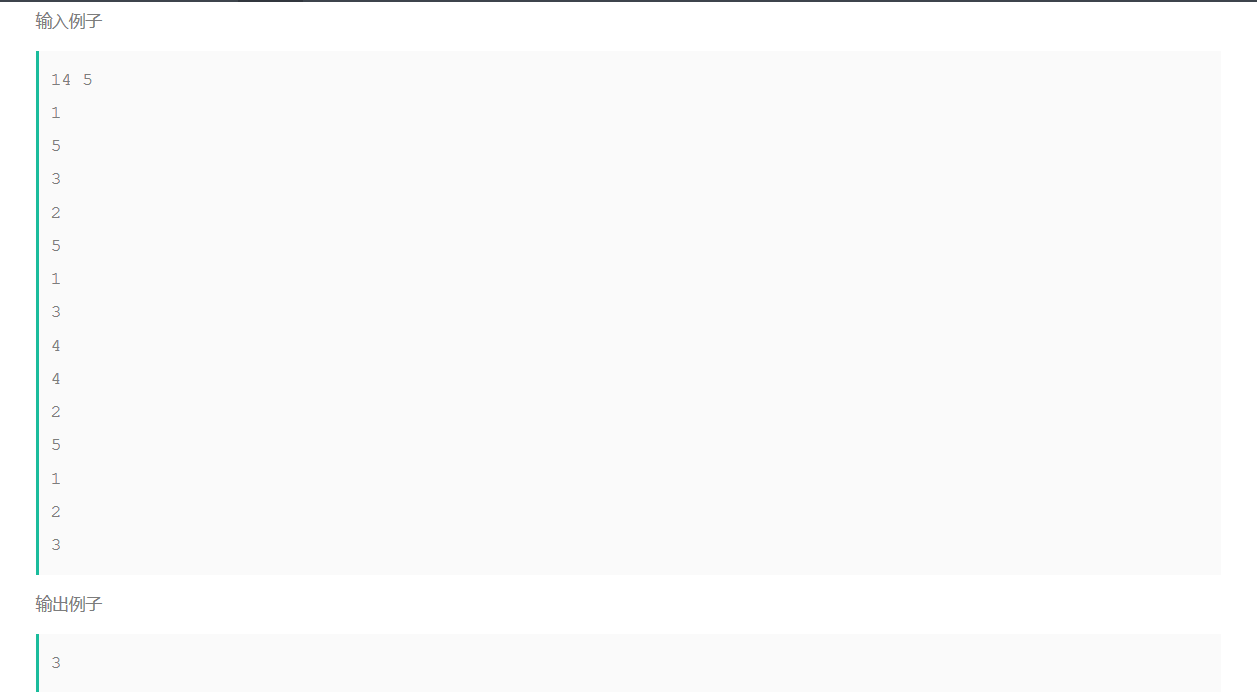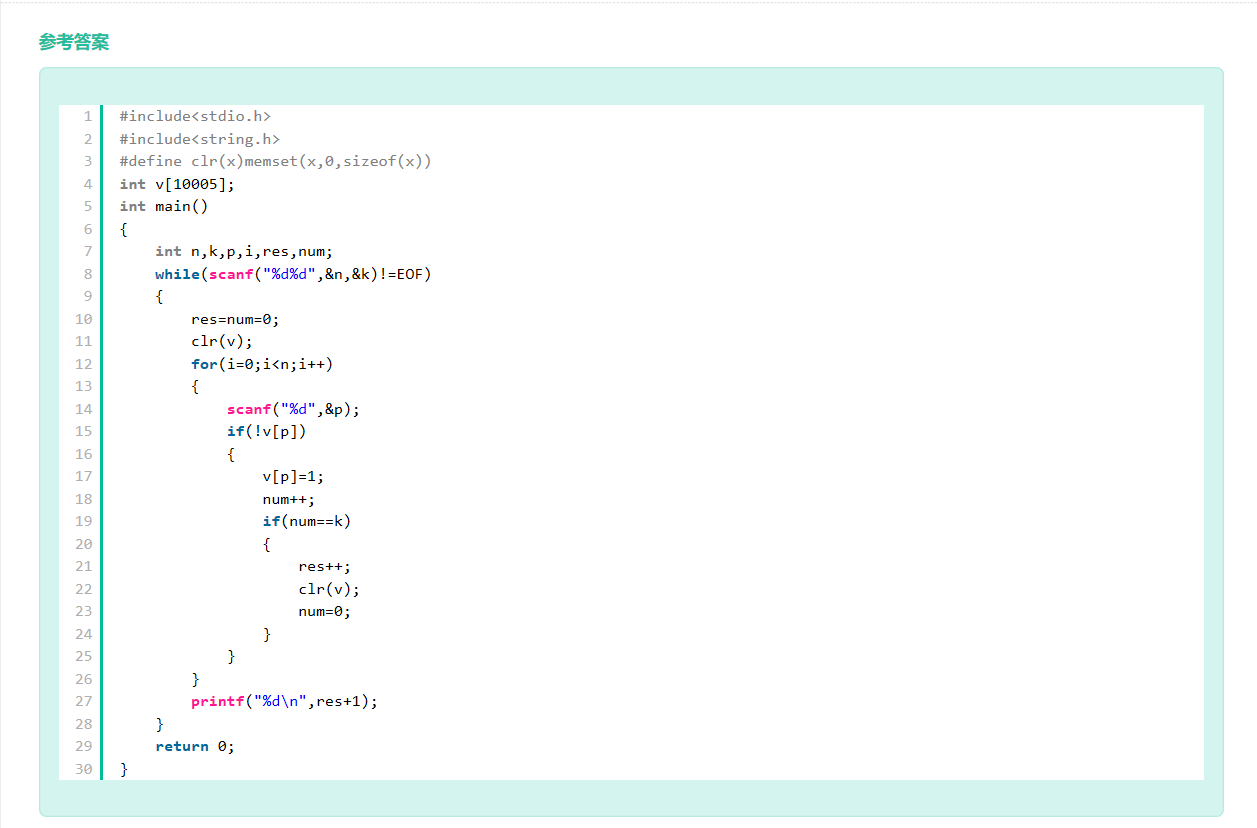• while循环条件那条语句使用的是EOF，关于这个EOF我也是前不久才知道的。EOF是End Of File的意思，在C语言中定义的一个宏，用作文件结束标志。从数值角度看，就是-1。这一条是我们可以学习的。
• "#define clr(x)memset(x,0,sizeof(x))",这一条语句很特别，它的常量定义不像我们是直接一个数，该代码是直接宏定义，这样做可以不浪费数字。值得学习。
• 整个代码给人一种很舒服的感觉，虽然可能看不懂代码的意思，但是代码本身的可读性还是很高的，这一点是林老师是第一堂课就强调的。
• 代码的数组变量是全局变量。我们好像不敢去用数组的全局变量，害怕会崩盘，一旦发生错误可能会导致整个程序崩溃。但是数组的全局变量在某类题目上是很有用的，这就需要我们分辨是否选择全局变量了。但是我们还是要学习优秀代码的数组的全局变量。
posted @ 2019-11-17 22:49  网络1911黄嘉欣  阅读(550)  评论(0编辑  收藏  举报
levels of contents